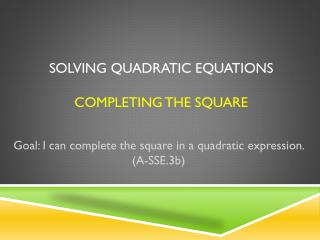DownloadDownload PresentationSolving Quadratic Equations Completing the Square

# Solving Quadratic Equations Completing the Square

Télécharger la présentation## Solving Quadratic Equations Completing the Square

- - - - - - - - - - - - - - - - - - - - - - - - - - - E N D - - - - - - - - - - - - - - - - - - - - - - - - - - -
##### Presentation Transcript

1. Solving Quadratic EquationsCompleting the Square Goal: I can complete the square in a quadratic expression. (A-SSE.3b)

2. Recall: Square Root Property • Solve the equation using the square root property.

3. Recall: Square Root Property • Solve the equation using the square root property.

4. To solve by Completing the Square • Step 1: Move the constant • Step 2: Add to each side. • Step 3: Factor the perfect square trinomial. • Step 4: Solve for x, using the Square Root Property.

5. Example 1: • Step 1: Move the constant. • What would you do to each side to move the constant?

6. Example 1: • Step 2: Add to each side.

7. Example 1: • Step 3: Factor the perfect square trinomial.

8. Example 1: • Step 4: Solve for x, using the Square Root Property.

9. Example 2: • Solve by completing the square.

10. Example 3: • Solve by completing the square.